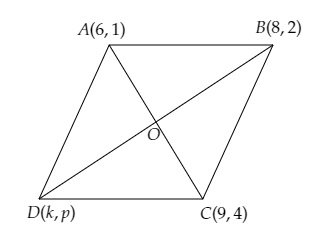# If the points $A (6, 1), B (8, 2), C (9, 4)$ and $D (k, p)$ are the vertices of a parallelogram taken in order, then find the values of $k$ and $p$.

Given:

The points $A (6, 1), B (8, 2), C (9, 4)$ and $D (k, p)$ are the vertices of a parallelogram taken in order.

To do:

We have to find the values of $k$ and $p$.

Solution:

Let the diagonals $AC$ and $BD$ bisect each other at $O$.Using the mid-point formula, we get,

$\mathrm{O}$ is the mid-point of $\mathrm{AC}$

The coordinates of $\mathrm{O}$ are $\left(\frac{6+9}{2}, \frac{1+4}{2}\right)$

$=(\frac{15}{2}, \frac{5}{2})$

Similarly,

$\mathrm{O}$ is the mid-point of $\mathrm{BD}$.

The coordinates of $\mathrm{O}$ are $\left(\frac{8+k}{2}, \frac{2+p}{2}\right)$

On comparing, we get,

$\frac{8+k}{2}=\frac{15}{2}$

$\Rightarrow 8+k=15$

$\Rightarrow k=15-8=7$

$\frac{2+p}{2}=\frac{5}{2}$

$\Rightarrow 2+p=5$

$\Rightarrow p=5-2=3$

The values of $k$ and $p$ are $7$ and $3$ respectively.

Updated on: 10-Oct-2022

88 Views